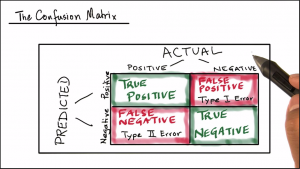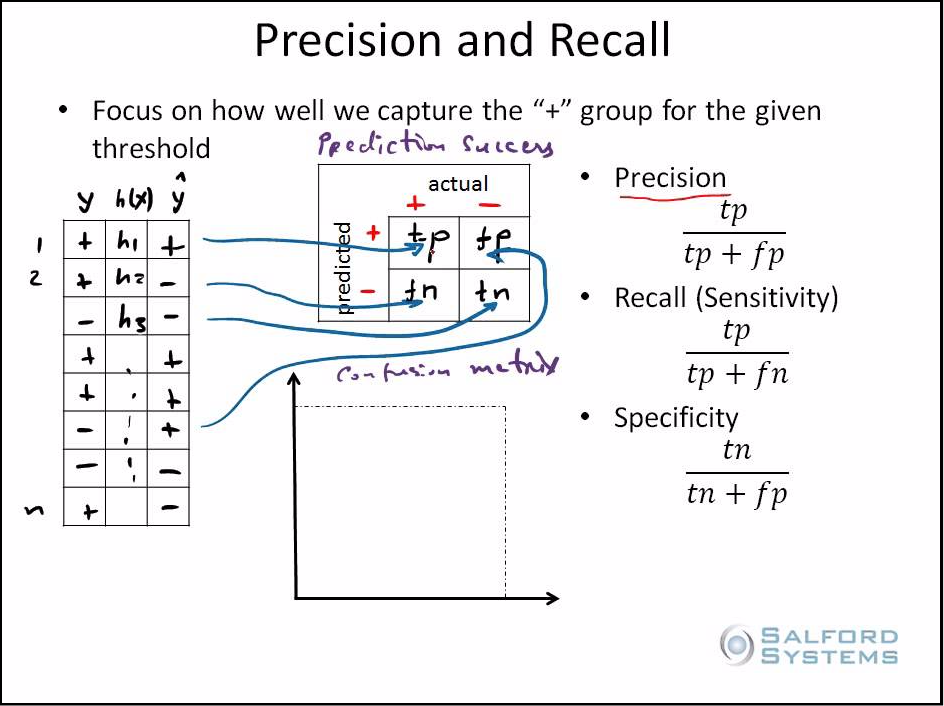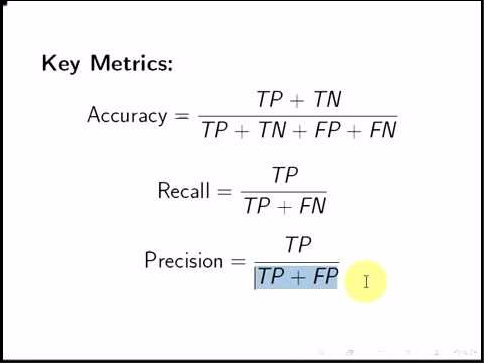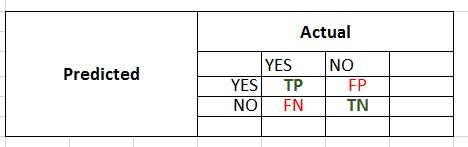## Model Prediction Success: Accuracy Vs Precision

The success of prediction model is calculated based on how well it predicts the target variable or label for the test dataset.

In a binary classification problem the label has two possible outcomes; for example, a classifier that is trained on patient dataset to predict the label 'disease' with values 'YES', 'NO' indicating presence of the disease or not. The prediction result for the label can be summarized in a confusion matrix to compare predicted values with actual values and from these values different measures are calculated to determine the predictive power of the classifier.## Confusion MatrixLabel - Disease

Binary Values - YES, NO

TP = True Positives - YES classified as YES

TN = True Negatives - NO classified as NO

FP = False Positive - NO classified as YES

FN = False Negative -  YES classified as NO

Predicted Positives
First Row Total  = TP + FP

Actual Positives
First Column Total = TP + FN

Predicted Negatives
Second Row Total = TN +  FN

Actual Negatives
Second Column Total = TN + FP

Prediction Measures

Predictions are correct when the predicted values match the actual values of the test dataset label. The diagonal values in the confusion matrix are the matching values and hence indicate the correct predictions.

The non-diagonal values of the confusion matrix are the mis-match between actual and predicted values of the test dataset label; and hence indicate the mis-classifications.

### Correct Predictions:

#### True Positives (TP)

The trained model predicts the patient has the disease and the patient actually has the disease. That is predicted values 'YES' for the label 'disease' match with the actual values 'YES' of the test data.

#### True Negatives (TN)

The trained model predicts the patient does not have the disease and the patient is actually disease free. That is predicted values 'NO' for the label 'disease' match with the actual values 'NO' of the test data.

### Mis-classifications:

#### False Positives (FP) - Type I error

The trained model predicts the patient has the disease but the patient is actually disease free. That is predicted values 'YES' for the label 'disease' do-not-match with the actual values 'NO' of the test data.
This is also known as Type I error wrt the null hypothesis.

#### False Negative (FN) - Type II error

The trained model predicts the patient does not have the disease but the patient actually has the disease. That is predicted values 'NO' for the label 'disease'  do-not-match with the actual values 'YES' of the test data.
This is also known as Type II error wrt the null hypothesis.

Based on these four values of the confusion matrix below metrics are used to measure the predictive power of the binary classifiers:

### Accuracy: Correct Predictions on Total

Proportion of correct classifications overall. That is total correct predictions over total observations?### Error Rate: Incorrect Predictions on Total

Proportion of incorrect predictions overall? That is total incorrect predictions over total observations.

Error = 1 - Accuracy### Precision: Correct Positive Predictions over Total Positive Predictions

How many of the 'YES' predictions are correct from the total 'YES' predictions?### Recall (Sensitivity): Correct Positive Predictions over Actual Positive Values

How many of the 'YES' predictions are correct from the actual 'YES' values (i.e. columns total)?### Specificity: Correct Negative Predictions over Actual Negative Values

How many of the 'NO' predictions are correct from the actual 'NO' values (i.e. second column total)?## Binary Classification Example

We classify the sale of child car seats as high or low and use confusion matrix to calculate the accuracy, precision, recall and specificity of the model.

## Install and Load required R packages

install.packages("ISLR")
install.packages("tree")
libs = c("ISLR","tree")
lapply(libs, require, character.only=TRUE)

## Understanding the Carseats dataset

We will use Carseats dataset from ILSR library.

The binary classification problem is to predict the Sales as high or low using remaining 10 variables as predictors for the classifier.

# see description of Carseats dataset

?Carseats

# Carseats {ISLR} R Documentation
# Sales of Child Car Seats

# Description

# A simulated data set containing sales of child car seats at 400 different stores.

# A data frame with 400 observations on the 11 variables.

# check dimensions of data set

dim(Carseats)
# 400 11

names(Carseats)
#  "Sales" "CompPrice" "Income" "Advertising"
#  "Population" "Price" "ShelveLoc" "Age"
#  "Education" "Urban" "US"

str(Carseats)

# 'data.frame': 400 obs. of 11 variables:
# \$ Sales : num 9.5 11.22 10.06 7.4 4.15 ...
# \$ CompPrice : num 138 111 113 117 141 124 115 136 132 132 ...
# \$ Income : num 73 48 35 100 64 113 105 81 110 113 ...
# \$ Advertising: num 11 16 10 4 3 13 0 15 0 0 ...
# \$ Population : num 276 260 269 466 340 501 45 425 108 131 ...
# \$ Price : num 120 83 80 97 128 72 108 120 124 124 ...
# \$ ShelveLoc : Factor w/ 3 levels "Bad","Good","Medium": 1 2 3 3 1 1 3 2 3 3 ...
# \$ Age : num 42 65 59 55 38 78 71 67 76 76 ...
# \$ Education : num 17 10 12 14 13 16 15 10 10 17 ...
# \$ Urban : Factor w/ 2 levels "No","Yes": 2 2 2 2 2 1 2 2 1 1 ...
# \$ US : Factor w/ 2 levels "No","Yes": 2 2 2 2 1 2 1 2 1 2 ...

## Pre-processing the data set

Target variable Sales is a continuous variable, since we cannot do classification on continuous variable  we create a new variable Sales_High to indicate sales is high or not.  If Sale value is greater than median we assign categorical value 'High' otherwise 'Low'

We look at the statistics of Sales and set a condition based on the range and median value to assign values to new variable Sales_High

# data manipulation to set condition for new variable

range(Carseats\$Sales)
# 0.00 16.27

summary(Carseats\$Sales)
# Min.     1st Qu.  Median  Mean   3rd Qu.  Max.
# 0.000  5.390    7.490      7.496   9.320     16.270

# create categorical variable to indicate high sales

Sales_High = ifelse(Carseats\$Sales >= 8, "High", "Low")

# now we can remove the first column 'Sales'

Carseats_new = Carseats[,-1]

dim(Carseats_new)
#  400 10

# append categorical variable 'Sales_High'

Carseats_new = cbind(Carseats_new[],Sales_High)

dim(Carseats_new)
#  400 11

names(Carseats_new)
#  "CompPrice" "Income" "Advertising" "Population"
#  "Price" "ShelveLoc" "Age" "Education"
#  "Urban" "US" "Sales_High"

## Set seed

To make result reproducible

set.seed(2)

## Create train and test data set

Split the data set in to two equal parts:

Training dataset as 2/3rd of dataset and Test dataset as remaining 1/3rd.

Use sample function to generate random numbers for indexes of dataset for training dataset and minus those indexes for testing dataset.

# generate random numbers for train index

train = sample(1:nrow(Carseats_new),                 nrow(Carseats_new)*2/3)

str(train)
# int [1:266] 74 281 229 67 374 373 51 328 184 216 ...

train
#  74 281 229 67 374 373 51 328 184 216 391 93 296 70 157
#  329 375 87 170 29 252 147 317 57 131 392 56 134 359 50
#  4 61 299 319 189 398 308 104 242 55 354 107 42 59 337
#  283 346 124 177 285 3 6 238 323 96 399 271 340 210 243
#  262 301 212 88 289 379 130 154 73 22 91 103 14 369 60
# and so on

test = -train

str(test)
# int [1:200] -74 -281 -229 -67 -374 -373 -51 -328 -184 -216 ...

test
#  -74 -281 -229 -67 -374 -373 -51 -328 -184 -216 -391 -93
#  -296 -70 -157 -329 -375 -87 -170 -29 -252 -147 -317 -57
#  -131 -392 -56 -134 -359 -50 -4 -61 -299 -319 -189 -398
#  -308 -104 -242 -55 -354 -107 -42 -59 -337 -283 -346 -124
#  -177 -285 -3 -6 -238 -323 -96 -399 -271 -340 -210 -243
# and so on

training_data = Carseats_new[train,]
testing_data = Carseats_new[test,]
testing_High = Sales_High[test]

dim(training_data)
#  266 11

dim(testing_data)
#  134 11

## Fit the tree model

The model is built using training dataset and the predictor depends on all features.

# fit the tree model using training data

tree_model = tree(Sales_High~., training_data)

# use the model to predict test data

test_pred = predict(tree_model, testing_data, type="class")

## Plot the Confusion Matrix

True Positives (TP) = 35
True Negatives (TN) = 68
False Positives (FP) = 10
False Negatives (FN) = 21

Predicted Positives = 35 + 10 = 45
Predicted Negatives = 21 + 68 = 89
Actual Positives = 35 + 21 = 56
Actual Negatives = 10 + 68 = 78

Total Observations = 134 = size testing dataset

# plot the confusion matrix

table(test_pred,testing_High)

#                         testing_High
#      test_pred           High    Low
#                           High    35      10
#                           Low     21       68

## Calculating Model Prediction Success

Accuracy

Error Rate

Precision

Recall

Specificity

# confusion matrix values

TP = 35
TN = 68
FP = 10
FN = 21

# row and column totals

Pred_Positives = TP + FP
Pred_Positives
# 45

Pred_Negatives = TN + FN
Pred_Negatives
# 89

Actual_Positives = TP + FN
Actual_Positives
#  56

Actual_Negatives = TN + FP
Actual_Negatives
#  78

Total_test_data = TP + TN + FP + FN
Total_test_data
#  134

# Accuracy

Accuracy = (TP + TN)/Total_test_data
Accuracy
# 0.7686567

# Error_rate

Error_rate = (FP + FN)/Total_test_data
Error_rate
#  0.2313433

Error_rate = 1- Accuracy
Error_rate
#  0.2313433

# Precision

Precision = TP/Pred_Positives
Precision
#  0.7777778

# Recall_Sensitivity

Recall_Sensitivity = TP/Actual_Positives
Recall_Sensitivity
#  0.625

# Specificity

Specificity = TN/Actual_Negatives
Specificity
#  0.8717949